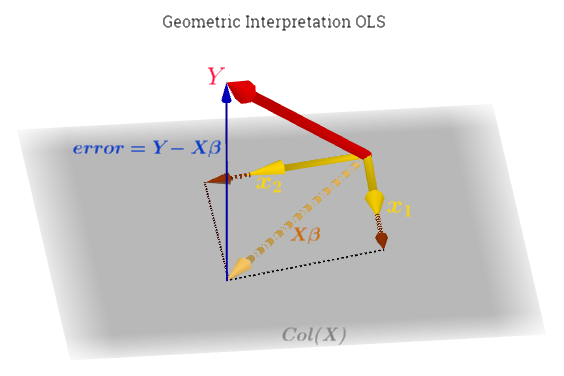### GEOMETRIC INTERPRETATION OF OLS:In the linear model $$y = \beta_0 + \beta_1 x_1 + \beta_2 x_2 + ... \beta_n x_n +\varepsilon$$ projection is key in the linear algebra of the model.

Why project? Because $$\mathbf{X} \mathbf{\beta} = \mathbf{y}$$ may have no solution if $$\mathbf{y}$$ it is not in the column space of $$\mathbf{X}$$. So instead we solve

$$\mathbf{X\,\hat \beta}= \mathbf{\hat y}\small \tag 1$$

where $$\mathbf{\hat y}$$ is the projection of the original outcome $$\mathbf{y}$$ on the column space of the regressors $$\mathbf{X}$$, or $$\mathscr C (\mathbf{X})$$.

The key is to see that $$\Large \mathbf{y} - \mathbf{X\hat \beta}=\varepsilon$$ is the error, and is perpendicular to $$c(\mathbf{X})$$.

Therefore, $$\mathbf{X}^T (\mathbf{y} - \mathbf{X\hat \beta}) =0 \small \tag 2$$

because we are dotting every vector in the $$Col(\mathbf{X})$$ with the error vector, and the errors are orthogonal. In other words, the error $$\mathbf{e}$$ is in the null space of $$\mathbf{X}^T$$.

Working on equation (2) we get to the NORMAL EQUATIONS (they are called the normal equations because they specify that the residual must be normal (orthogonal) to every vector in the span of $$A$$:

$$\color{blue}{\mathbf{X}^T \mathbf{X\,\hat \beta} = \mathbf{X}^T \mathbf{y}} \quad \text{ NORMAL EQUATIONS}\tag { 3}$$

INTERLUDE:

What does $$X^\top X$$ look like if we consider only one regressor (in blue): $$x_{i1}$$?

\begin{align} X^\top X &= \begin{bmatrix} \color{blue}{1} & \color{blue}{1} & \color{blue}{1} &\color{blue}{\cdots}\\ \color{blue}{x_{11}} & \color{blue}{x_{21}} & \color{blue}{x_{31}} &\color{blue}{\cdots}\\ x_{12} & x_{22} & x_{32} &\cdots\\ \vdots & \vdots & \vdots &\ddots \end{bmatrix}\begin{bmatrix} \color{blue}{1} & \color{blue}{x_{11}} & x_{12} & \cdots\\ \color{blue}{1} & \color{blue}{x_{21}} & x_{22} & \cdots\\ \color{blue}{1} & \color{blue}{x_{31}} & x_{32} & \cdots\\ \color{blue}{\vdots} & \color{blue}{\vdots} & \vdots & \ddots \end{bmatrix} \\[2ex] \underset{\text{blue elements only}}{=}&\quad\color{blue}{\begin{bmatrix}n & \sum x_{i1}\\ \sum x_{i1} & \sum x_{i1}^2\end{bmatrix}} \end{align}

Obtaining the determinant of this matrix:

$$det(X^\top X) = n \sum x_{i1}^2 - \left[ \sum x_{i1} \right]^2 \underset{*}{=}n\, \sum (x_{i1}-\bar x)^2$$

$$(*)$$ since the shortcut formula for the variance is $$\sigma^2 = \frac{\sum (x-\mu)^2}{n}=\frac{n\,\left(\sum x^2\right) - \left(\sum x\right)^2}{n^2}$$

Leading to the formula for $$(X^\top X)^{-1}$$

\begin{align} (X^\top X)^{-1} &= \frac{1}{n\, \sum (x_{i1}-\bar x)^2}\color{blue}{\begin{bmatrix}\sum x_{i1}^2 & -\sum x_{i1}\\ -\sum x_{i1} & n\end{bmatrix}}\\[2ex] &\underset{\text{simpler indices}}{=} \begin{bmatrix}\frac{\sum x_i^2}{n\sum(x_i - \bar x)^2} & \frac{-\sum x_i}{n\sum(x_i - \bar x)^2}\\\frac{-\sum x_i}{n\sum(x_i - \bar x)^2}& \frac{1}{\sum(x_i - \bar x)^2}\end{bmatrix} \end{align}

Isolating $$\mathbf{\hat \beta}$$:

$$\left(\mathbf{X^TX}\right)^{-1} \, \mathbf{X}^T \mathbf{y} = \mathbf{\hat \beta} \small \tag 4$$

Plugging these results into equation (1):

$$\color{red}{\mathbf{X}\,\left(\mathbf{X^TX}\right)^{-1} \, \mathbf{X}^T\,\,\, \mathbf{y} =\mathbf{X}\,\mathbf{\hat \beta}= \mathbf{\hat y}} \tag 5$$

The projection matrix (which the same as the hat matrix) is defined as:

$$\color{blue}{\mathbf{H}= \mathbf{X} \left(\mathbf{X}^{T} \mathbf{X} \right)^{-1} \mathbf{X} ^{T}} \text{ PROJECTION MATRIX} \tag 6$$

because it put a “hat” on the $$\mathbf{y}$$. It is both symmetrical and idempotent, as it corresponds to a projection matrix.

Its orthogonal complement, also referred to as the annihilator, is:

$$\mathbf{M}= \mathbf{I}_n- \mathbf{H} \small \tag 7$$

$$n$$ representing the number of rows and columns of $$\mathbf{H}$$.

#### Properties:

$$\mathbf{H}\,\,\mathbf{X} = \mathbf{X}$$

$$\mathbf{M}\,\,\mathbf{X} = \left(\mathbf{I}_n- \mathbf{H}\right)\,\mathbf{X}= \mathbf{X}- \left(\mathbf{H}\,\mathbf{X}\right)$$

The residuals can be represented as:

$$\mathbf{e}=\mathbf{M}\mathbf{y} =\mathbf{y}- \mathbf{H}\mathbf{y}= \mathbf{y}-\mathbf{\hat y}$$

The loss function (objective function) that is minimized is the sum of the squared residuals:

$$f(\beta) = \bf (y - X\beta)^\prime(y - X\beta) \text{ OBJECTIVE FUNCTION}$$

### Random vectors:

$$\mathbf{X}$$ are considered fixed measurements without error, whereas the following are random vectors:

$$\mathbf{\hat y}$$ (fitted values), $$\hat \beta$$ (betas) and $$\mathbf{e}$$ (residuals).

since the two of them are linear combinations of the random vector $$\mathbf{y}$$.